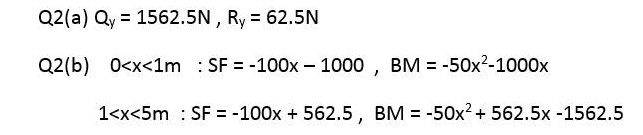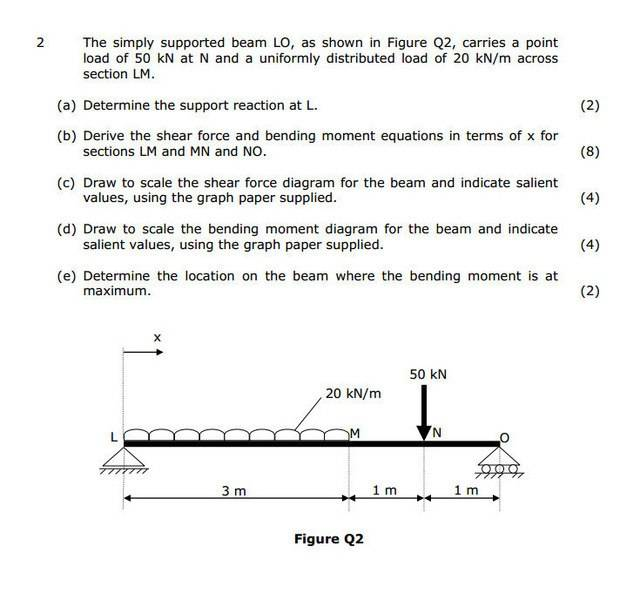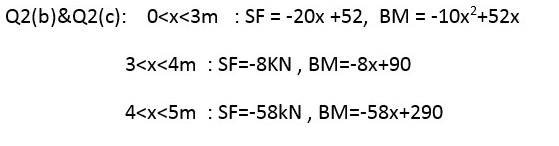# Shear Force/Bending Moments Equation

Hi, question and solution attached.

I'm having trouble converting the second equation in 2 (b) to the corresponding bending moment equation. I have worked out everything else, I'm just unsure how the '-1562.5N' part is calculated.

http://snag.gy/Uqrel.jpgHelp is much appreciated, thanks!

Doesn't the first equation for BM have to equal the second equation BM at the same point Q?

Doesn't the first equation for BM have to equal the second equation BM at the same point Q?

So because Q is 1562.5 it is taken away from the second BM equation?

PhanthomJay
Homework Helper
Gold Member
The integral of the shear force is the bending moment. -1562.5 is the constant of integration. Why?

The integral of the shear force is the bending moment. -1562.5 is the constant of integration. Why?

I'm not understanding how that constant is reached in this instance.

PhanthomJay
Homework Helper
Gold Member
I'm not understanding how that constant is reached in this instance.between x =1 and x=5, if you are good with the SF, then the BM equation is the integral of it, that is,
BM = -50x^2 + 562.5x + constant

(you're ok with that?)

To solve for the constant, see paisiello2 hint in post 2. Or note that the bending moment is ??? at x=5.between x =1 and x=5, if you are good with the SF, then the BM equation is the integral of it, that is,
BM = -50x^2 + 562.5x + constant

(you're ok with that?)

To solve for the constant, see paisiello2 hint in post 2. Or note that the bending moment is ??? at x=5.

Yeah I get that.

Ok, so you just sub 5 into x. Why is the constant not present in the first bending moment equation though?

Also, in terms of this example here, just looking at the second equation in 2. (b), why is the constant not applied in the same way when integrating the second SF equation? It is in the 3rd, but not the second.Sorry for the amount of questions, but I'm quite confused by this...

PhanthomJay
Homework Helper
Gold Member
Yeah I get that.

Ok, so you just sub 5 into x.
yes sub the 5 into x and set the bending moment equal to __?__ to solve for the constant.
Why is the constant not present in the first bending moment equation though?
It is, but it happens to be zero

PhanthomJay
Homework Helper
Gold Member
Also, in terms of this example here, just looking at the second equation in 2. (b), why is the constant not applied in the same way when integrating the second SF equation? It is in the 3rd, but not the second.Sorry for the amount of questions, but I'm quite confused by this...
If you are having trouble determining the constant of integration, it is probably best to draw free body diagrams at a cut in the sections to determine the BM in that section, using the equilibrium equations. For better assistance, please show your workings in determining the shear force and moment equations in one of the sections.

If you are having trouble determining the constant of integration, it is probably best to draw free body diagrams at a cut in the sections to determine the BM in that section, using the equilibrium equations. For better assistance, please show your workings in determining the shear force and moment equations in one of the sections.

Ok, I'll give you two examples to illustrate where I'm seeing a lack of consistency.

In the second BM equation in the first example I provided after integrating the SF equation you get this...

-50x^2 + 562.5x + C
C = 50 x 5^2 - 562.5 x 5
= - 1562.5
BM = -50x^2 + 562.5x - 1562.5

That's the correct solution as shown in the answers provided and as you've explained.

Now again looking at the second BM but this time in the second example provided after SF is integrated you get...

-8x + C
C = 8x
= 8 x 5
= 40

That's incorrect, as shown in the solution C = 90.

So what am I missing out here?

I notice that if I carry out the same method on the third equation it does again provide the correct answer, it's just that last example I'm not understanding. I could do something with the 50 at 4m that would get an answer of 90 but that wasn't necessary in the other two equations.

PhanthomJay
Homework Helper
Gold Member
Ok, I'll give you two examples to illustrate where I'm seeing a lack of consistency.

In the second BM equation in the first example I provided after integrating the SF equation you get this...

-50x^2 + 562.5x + C
you mean BM = -50x^2 + 562.5x + C , then set BM = 0 at x =5 (pinned support), to solve for C.
C = 50 x 5^2 - 562.5 x 5
= - 1562.5
BM = -50x^2 + 562.5x - 1562.5
ok
That's the correct solution as shown in the answers provided and as you've explained.

Now again looking at the second BM but this time in the second example provided after SF is integrated you get...

-8x + C
C = 8x
= 8 x 5
= 40

That's incorrect, as shown in the solution C = 90.

So what am I missing out here?
The BM is -8x +C between x =3 and x=4, so you can't solve for the constant at x =5. You would have to determine the BM at x=4 from the third equation, then use that value (BM = 58) when writing -8X + C = 58 at x = 4, solve for C.
I notice that if I carry out the same method on the third equation it does again provide the correct answer, it's just that last example I'm not understanding. I could do something with the 50 at 4m that would get an answer of 90 but that wasn't necessary in the other two equations.
Again, using Free Body Diagrams to determine the BM equations is an alternate method.